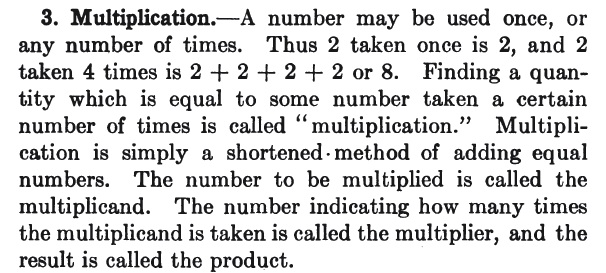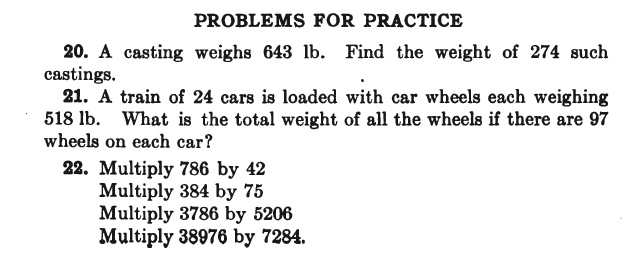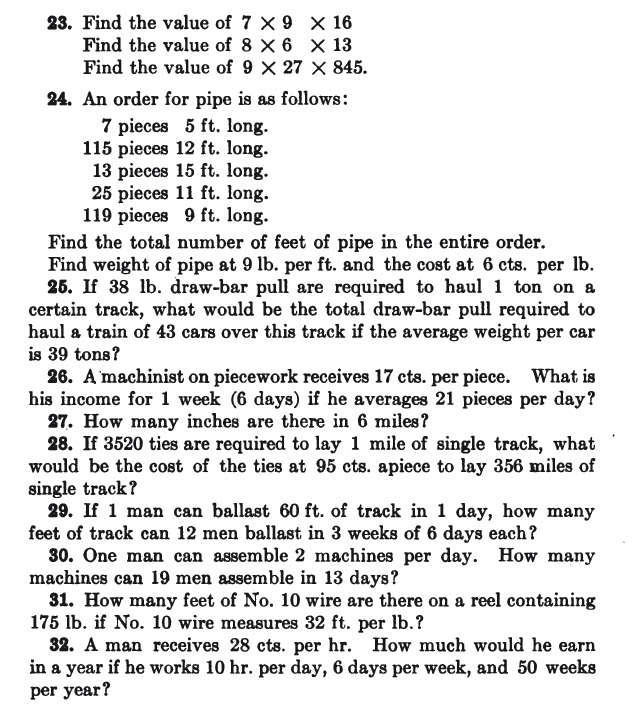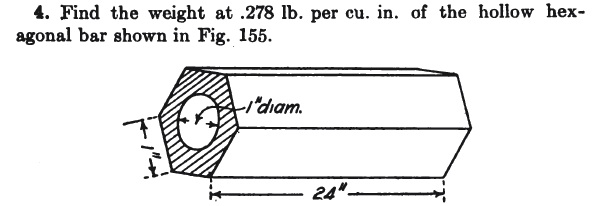# Practical Applied Mathematics (wherein multiplication is treated as repeated addition)

Posted by: Gary Ernest Davis on: January 6, 2011

She wrote:

When I was in school, I was having problems with algebra and he (Hale) tutored me and gave me a book he had written, and signed, which was titled “Practical Applied Mathematics.” I still have the book, which was published in 1915, and I cherish it.

How wonderful that the author remembered with fondness a book on applied mathematics, and algebra, after so many years.

When I mentioned this story on Twitter John Rowland (@SofARMaths)tweeted:

Wonder if I can still get a second hand copy of Practical Applied Mathematics 1915…

I checked in Google books and sure enough, the entire book, published originally by the McGraw-Hill Book Company Inc. in 1915, is available for download as a pdf: Practical_applied_mathematics_Hale

The author wrote the book for trade and vocational schools, building on his experience with railroad schools. The book is intended to present mathematics as a useful tool to trade students.

Early in the book – page 5 to be exact – Hale introduces multiplication of whole numbers. Here is what he writes:It is clear from this passage that Hale regards multiplication as simply shorthand for the process of repeatedly adding a given number: in short, as repeated addition.

What, if anything, does this illustrate or prove?

I think it proves nothing, but in the context of the entire book it illustrates something important: Hale was seeking a direct approach to mathematical topics so that his trade school students could use the mathematics as a tool. He did not introduce wrong thinking, or wrong methods of calculation, but often simply cut to the chase as if to say “this is how it is”.

Hale sets some multiplication problems as exercises. Some of these are straight arithmetical problems, with no other context. But the majority of them are practical problems likely to be encountered by trade students. These are not contrived problems, such as one often sees nowadays in textbooks, problems that pass for practical problems but are simply artificial situations invented by an author who knows little of the applications of mathematics.Hale gives approximate rules for many calculation. For example he introduces$\pi$ as 3.1416 and he states the areas of polygons as a set of rules, without explanation. In this regard, his practice is not that much different from contemporary mathematics teachers.

He introduces some beautiful geometric problems in a practical trade setting:Hale’s book is, in my view, a rich source of ideas and problems for all students of mathematics. He treats multiplication of positive whole numbers explicitly as repeated addition and that seems to work well for his purposes.

This does not mean multiplication SHOULD be taught as repeated addition, and certainly not that it should be taught only as repeated addition.

Hale’s book, as good as it is for the purpose he intended, is quite old and does not take into account many years in between of knowledge of how students learn mathematics (but, to be fair, neither do most contemporary text books, which are differentiated from Hale’s mainly in their exorbitant cost).

To the best of my knowledge no one has yet to come up with a model for multiplication that acts as a cognitive root –  a way of thinking about a concept that, while not formally correct, nor complete in all details, is sufficiently powerful to allow students to use the concept, and to not have to throw their ideas overboard as their learning advances:

“A cognitive root is a concept that:
(i) is a meaningful cognitive unit of core knowledge for the student at the beginning of the learning sequence,
(ii) allows initial development through a strategy of cognitive expansion rather than significant cognitive reconstruction,
(iii) contains the possibility of long-term meaning in later developments,
(iv) is robust enough to remain useful as more sophisticated understanding develops.”

### 5 Responses to "Practical Applied Mathematics (wherein multiplication is treated as repeated addition)"I am wondering if you consider the two phrases, “multiplication is …” and “multiplication as …” different.

In the teacher’s edition of a Japanese elementary math textbook series, the authors stated not to think of multiplication as repeated addition as repeated addition is a method of calculating the product (just as something like skip counting is a method of calculating the product). Is it important (at least for teachers who are teaching young children) to distinguish what something “is” and how to actually carry it out? Another common example we see often of blurring of “is” and “how to” is the idea of arithmetic mean. A lot of students say that the mean “is” the sum of the numbers divided by the number of numbers, but is it?Yes, I would say the mean of the numbers$x_1\ldots x_n$ is$\frac{x_1+\ldots +x_n}{n}$.
What the mean “IS” gives us a procedure for calculating it.
At least that’s my take on it.

Your comments remind me of a presentation of Joop Vandermolen some years back. He was detailing student understanding of square roots. Generally, very broadly (and possibly incorrectly!) I recall Joop as saying that lower achieving mathematics students saw “square root” as something to do; the square root of 49 is 7 because when you square 7 you get 49. The high achieving mathematics students on the other hand generally saw square roots as objects: the square root of a number is another number whose square is the first.

I can say what I would think if asked what “IS”multiplication (it’s a certain bilinear function), but
I do not know what teachers and students mean by what multiplication “IS”. What do the Japanese texts say it is (or don’t they say) ?Most Japanese textbooks will introduce multiplication as the operation to find the total amount when you have so many of equal sized groups with so many elements in each. I guess they distinguish “multiplication is the operation…” from how to find the total amount. Perhaps just as you describe the mean, this definition suggests that *a* procedure to find the product is to use the repeated addition using the multiplicand as the addend. Also, most Japanese textbooks introduce more of a comparison idea before getting into learning multiplication facts. So, multiplication is also the operation to find the amount that is so many times as many as the given. This is probably similar to/in alignment with what Devlin calls “scaling.” Most Japanese textbooks then use this idea to extend multiplication (and division – division is the operation to find how many times as many [quotitive] or the original amount [partitive]) of decimal numbers then to fractions.I think you’ve raised a very good point: the difference between what “blah” IS, and how one calculate with “blah” – where in this case “blah” is whole number multiplication.

This might explain some of the amazingly emails I get about this topic – possibly people are confusing the two things?I really think this illustrates a point I made in an article on Associated Content (now Yahoo).